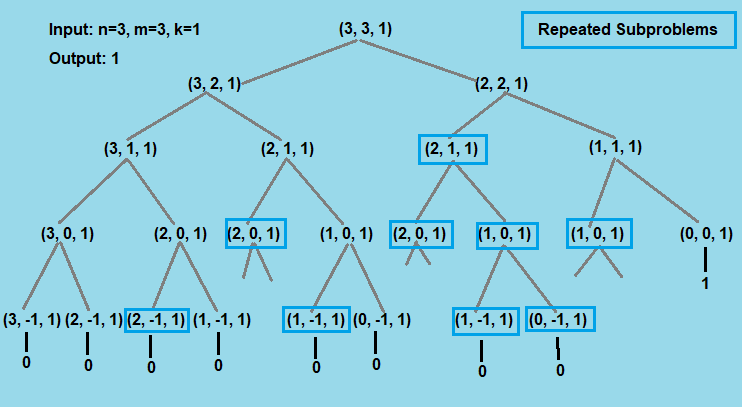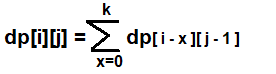×

Search anything:

# Tile Stacking Problem

#### Algorithms Dynamic Programming (DP) prefix sum arrayGet this book -> Problems on Array: For Interviews and Competitive Programming

Reading time: 40 minutes

A stable tower of height n is a tower consisting of exactly n tiles of unit height stacked vertically in such a way, that no bigger tile is placed on a smaller tile i.e. we can place a tile of size smaller than or equal to the last tile placed onto the stack. An example configuration is shown below :We have infinite number of tiles of sizes 1,2,3,...m. The task is calculate the number of different stable tower of height n that can be built from these tiles, with a restriction that you can use at most k tiles of each size in the tower.
So we have to find total number of different tile stacks using at most k tiles of each size.

We demonstrate how we will reduce the time complexity from O((k+1)m+1) to O(N * M * K) to O(N * M)

Note: Two towers of height n are different if and only if there exists a height h(1 <= h <= n), such that the towers have tiles of different sizes at height h.

Examples:

``````Input : n = 3, m = 3, k = 1.
Output : 1
Possible sequences: { 1, 2, 3}.
So the answer is 1.

Input : n = 3, m = 3, k = 2.
Output : 7
{1, 1, 2}, {1, 1, 3}, {1, 2, 2},
{1, 2, 3}, {1, 3, 3}, {2, 2, 3},
{2, 3, 3}.
So the answer is 7.
``````

Here we basically need to count the number of decreasing sequences of length n using the numbers from 1 to m where every number can be used at most k times. We can recursively compute count for n using count for n-1.
This problem can be efficiently solved by using dynamic programming. We will see two approaches one which is a naive approach(Brute force) and the other is a dynamic programming approach where we will reduce the time complexity from O((k+1)m+1) to O(n*m).

# Brute Force approach `:` O((k+1)m+1)

The naive solution for this problem is to generate all possible subproblems of the original problem and count the valid ones. Here we recursively solve the initial problem by checking if we can build a stack of hight n by using a particular tile i times(0 <= i <= k). So the problem is divided into smaller subproblems of the same type and solved recursively, at last we add up all the possible solutions of smaller subproblems to find the solution of the parent problem. Total uses of each tile is maintained by the stack overhead(stack data) of recursive calls so that it does not exceed k.
While going with the recursive approach, we go to each and every possible permutation and thus it takes O((k+1)m+1) time.

``````int tileStackingProblem(int n, int m, int k) {
if(m<0 || n<0)
return 0;
if(n==0)
return 1;
int res = 0;
for(int i=0;i<=k;i++){
res+=tileStackingProblem(n-i,m-1,k);
}
return res;
}
``````

Below is a tree representation of recursive calls of naive approach for the problem.Here, same sub-problems(Blue squared) are getting computed again and again. As we can see Tile Stacking Problem has optimal substructure and overlapping subproblems, it can be solved by Dynamic programming, where values can be Memoized or Tabulated rather than computing it again and again.

# Dynamic Programming approach : `O(n*m)`

In Dynamic Programming we declare a 2D array dp[][], where each state dp[i][j] denotes the number of decreasing sequences of length i using numbers from 1 to j. We need to take care of the fact that a number can be used a most k times. This can be done by considering 1 to k occurrences of a number. Hence our recurrence relation becomes:``````int dp;
// use memset(dp, -1, sizeof(dp)); in main to initialize.
int tileStackingProblem(int n, int m, int k) {
if(dp[n][m]!=-1)
return dp[n][m];
if(n==0)
return dp[n][m]=1;
if(m==0)
return dp[n][m]=0;
int res = 0;
for(int i=0;i<=k;i++){
res+=tileStackingProblem(n-i,m-1,k);
}
return dp[n][m]=res;
}
``````

The time complexity of the above solution is O(n*m*k). But, we can further improve it.
We can use the fact that for a fixed j we are using the consecutive values of previous k values of i. Hence, we can maintain a prefix sum array for each state. Now we have got rid of the factor k for each state. Hence the time complexity now reduces to O(n*m).
So we get the recurrence:

``````dp[i][j] = prefixsum[i][j - 1];
if (i > k)
{
dp[i][j] -= prefixsum[i - k - 1][j - 1];
}
``````

And prefix sum can be calculated by:

``````prefixsum[i][j] = prefixsum[i - 1][j] + dp[i][j];
``````

# Implementation

code in C++

``````// C++ program to solvse the Tile Stacking problem by DP
#include <iostream>
#include<cstring>

using namespace std;

long int tileStackingProblem(int n, int m, int k){
// in case of larger input data, declare the following arrays globaly.
// as global variables are declared in heap so it will get rid of memory overflow problem.
long int dp[n+1][m+1];
long int prefixsum[n+1][m+1];

memset(dp, 0, sizeof(dp));
memset(prefixsum, 0, sizeof(prefixsum));

// Initialing 0th column of dp to 0. i.e.
//If we have n left but m is 0, this means it is not possible to stack any more tile,
//thus setting it to 0
for(int i = 0; i < n + 1; i++)
{
dp[i] = 0;
prefixsum[i] = 1;
}

// Initialing 0th Row to 1. i.e.
//If we have n=0 left and m tiles remains, this means we successfully built a stable stack,
//thus setting it to 1
for(int i = 0; i < m + 1; ++i)
{
dp[i] = 1;
prefixsum[i] = 1;
}

// For each column from 1 to m
for (int j = 1; j < m + 1; j++)
{
// For each row from 1 to n.
for (int i = 1; i < n + 1; i++)
{
// Initialing dp[i][j] to presum of (i, j - 1).
dp[i][j] = prefixsum[i][j - 1];

if (i > k)
{
dp[i][j] -= prefixsum[i - k - 1][j - 1];
}
}

// Calculating prefixsum for each i, 1 <= i <= n.
for (int i = 1; i < n + 1; i++)
prefixsum[i][j] = dp[i][j] + prefixsum[i - 1][j];
}
return dp[n][m];
}

int main()
{
int n,m,k;
cin>>n>>m>>k;

cout<<"Tatal possible stable stacks = "<<tileStackingProblem(n ,m ,k)<<'\n';

return 0;
}
``````

# Complexity

Time Complexity

• Naive approach of the problem takes O((k+1)m+1) time.
• Dynamic Programming approach of the problem takes O(n*m) time.

Space Complexity

• Naive approach takes exponential(O(k+1)m+1) stack overhead and O(1) auxiliary space.
• Dynamic Programming takes O(n*m) auxiliary space.